### Home > CCAA > Chapter 1 CC2: Unit 1 > Lesson CC2: 1.2.2 > Problem1-74

1-74.

Read about scaling axes. Then, on your paper, copy the incomplete axes below and write the missing numbers on each one.

1.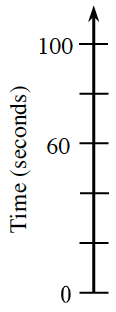Look at the two ticks labeled $60$ and $100$. What is the difference of $60$ and $100$? How many spaces are between them?

See the number line below.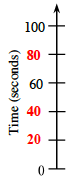1.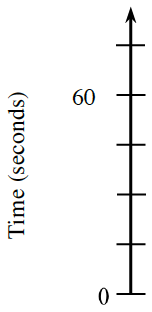What do you need to divide 60 by to get the number of spaces between each tick mark?

1.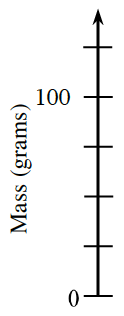What do you need to divide 100 by to get the number of spaces between each tick mark?

1.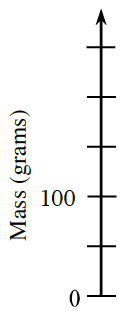See part (c).